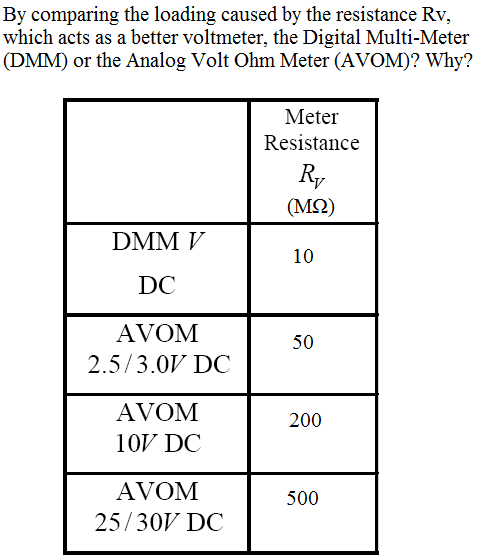# Voltmeter Problem

temaire

## Homework Statement## The Attempt at a Solution

I think that the AVOM meter would act as a better voltmeter. This is because it has a higher meter resistance, meaning that it is more sensitive. Therefore, it will disturb the circuit as little as possible and it will draw less current to operate compared to the DMM.

Gold Member
This is because it has a higher meter resistance...

Higher resistance is good, as when the meter is placed in parallel with the circuit, the very high parallel resistance will draw very low current, and thus not change the circuit operation in noticeable ways. Do some math with a simple circuit with a 1V source and a 1R resistance representing the circuit, and then put a 500MR resistance representing the meter in parallel and see how the current is split.

...meaning that it is more sensitive...

Do you mean that an AVOM gives more accurate readings than a DMM? Do you have an explanation or justification for this?

Therefore, it will disturb the circuit as little as possible...

I think this is in partnership with the first quote, though explaining 'disturb' shows a better understanding of the actual mechanics.

...and it will draw less current to operate compared to the DMM.

What power sources do AVOM and DMM use, and why does one draw less current to operate than the other?

temaire
Do you mean that an AVOM gives more accurate readings than a DMM? Do you have an explanation or justification for this?

I meant that an AVOM has a higher "ohm per volt" value.

I think this is in partnership with the first quote, though explaining 'disturb' shows a better understanding of the actual mechanics.

By "disturb" I meant that the AVOM meter will draw less current from the circuit.

What power sources do AVOM and DMM use, and why does one draw less current to operate than the other?

The DMM and AVOM used a DC power supply (this question is from a lab experiment). I think that the AVOM meter draws less current than the DMM because it has a higher resistance. More specifically, a voltmeter with a high resistance will not alter the resistance ratio of the voltage divider circuit as much.

So is my answer generally correct? Is it not specific enough? Thank you for your help.

Gold Member
So is my answer generally correct? Is it not specific enough? Thank you for your help.

Yes it is generally correct.

I think that the AVOM meter draws less current than the DMM because it has a higher resistance. More specifically, a voltmeter with a high resistance will not alter the resistance ratio of the voltage divider circuit as much.

I like this one better than the original!